# 逻辑回归--分类问题【机器学习】

## 问题引入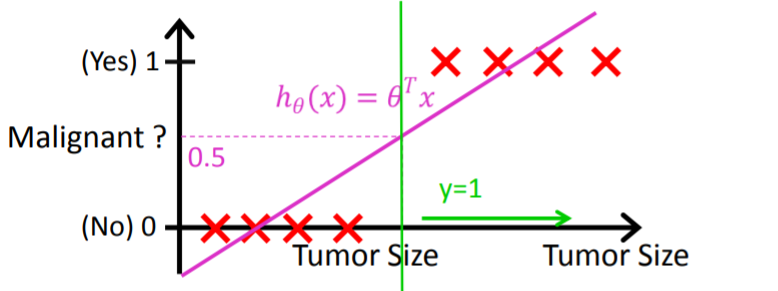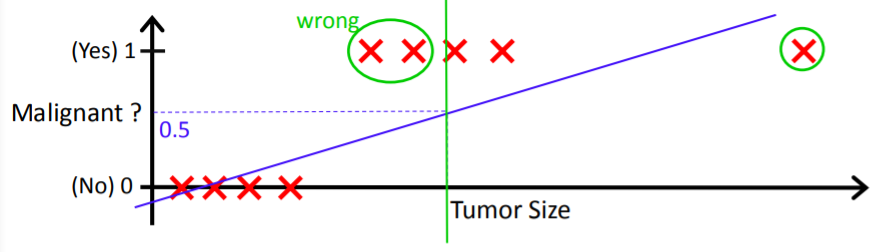## 逻辑回归

0 &lt; = h θ ( x ) &lt; = 1 0 &lt;= h_\theta(x) &lt;= 1

g ( z ) = 1 1 + e − z g(z) = \frac{1}{1 + e^{-z}}

h θ ( x ) = g ( θ T x ) = 1 1 + e − θ T x h_\theta(x) = g(\theta^Tx) = \frac{1}{1 + e^{-\theta^Tx}}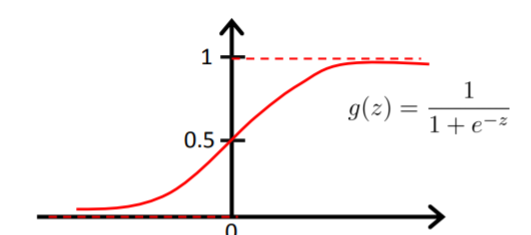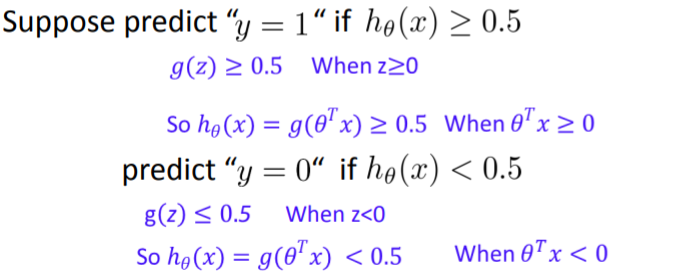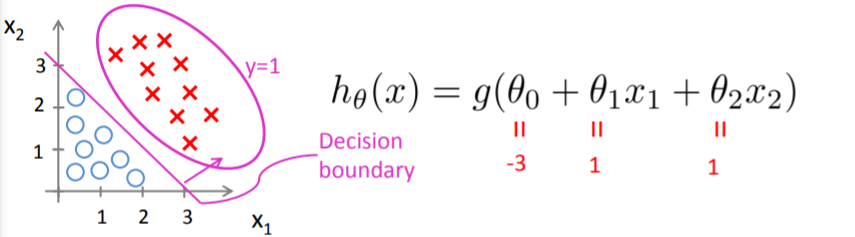## 逻辑函数的推导

y = 1 1 + e − z y = \frac{1}{1 + e^{-z}}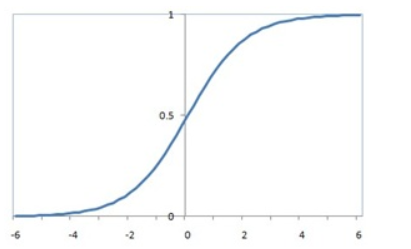P ( Y = 1 ∣ X ) = e z 1 + e z P(Y = 1|X) = \frac{e^z}{1 + e^z}
P ( Y = 0 ∣ X ) = 1 1 + e z P(Y = 0|X) = \frac{1}{1 + e^z}

l o g P ( Y = 1 ∣ X ) 1 − P ( Y = 1 ∣ X ) = z = &gt; p 1 − p = e z = &gt; 1 − p p = e − z = &gt; p = 1 1 + e − z log\frac{P(Y = 1|X)}{1 - P(Y = 1|X)} = z =&gt; \frac{p}{1 - p} = e^z =&gt; \frac{1 - p}{p} = e^{-z} =&gt; p = \frac{1}{1 + e^{-z}}

## 损失函数的设置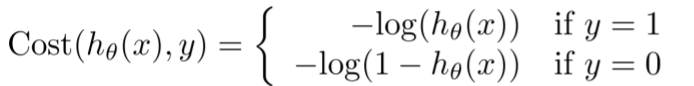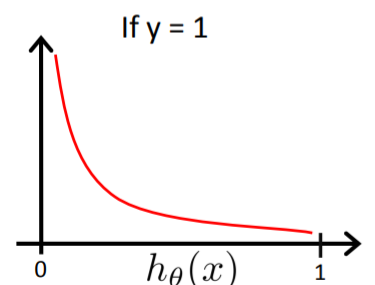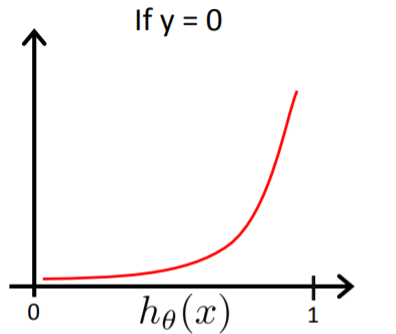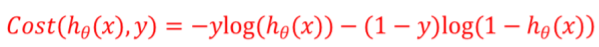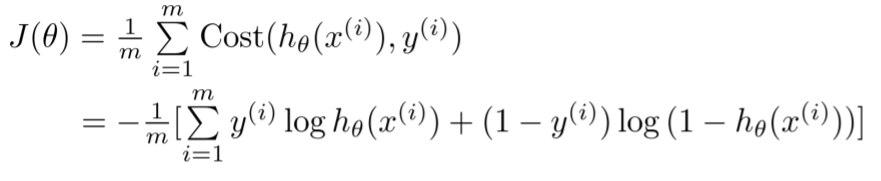## 梯度下降训练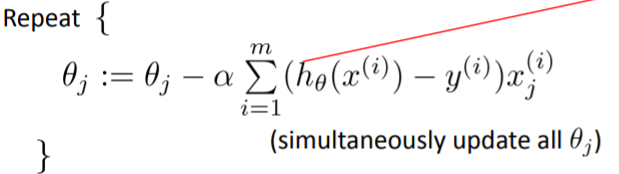## 延申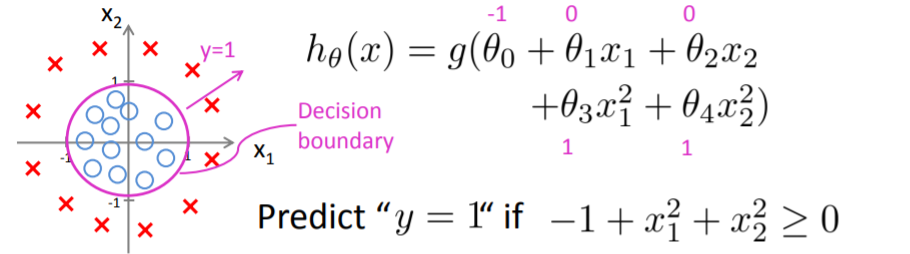05-316560
10-171395
03-287406
07-311480
07-1627万+
02-15573
09-0254
04-231011
04-30114